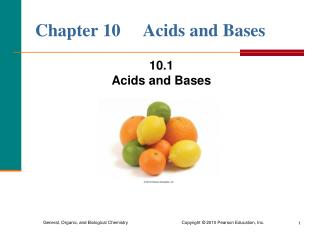# Chapter 10 Acids and Bases - PowerPoint PPT PresentationDownload PresentationChapter 10 Acids and Bases

Chapter 10 Acids and BasesDownload Presentation## Chapter 10 Acids and Bases

- - - - - - - - - - - - - - - - - - - - - - - - - - - E N D - - - - - - - - - - - - - - - - - - - - - - - - - - -
##### Presentation Transcript

1. Chapter 10 Acids and Bases 10.1Acids and Bases

2. Acids Arrhenius acids • produce H+ ions in water H2O HCl(g) H+(aq) + Cl(aq) • are electrolytes • have a sour taste • turn litmus red • neutralize bases

3. Names of Acids • Acids with H and a nonmetal are named with the prefix hydro- and end with -ic acid. HCl hydrochloric acid • Acids with H and a polyatomic ion are named by changing the end of the name of the polyatomic ion from -ate to -ic acid or -ite to -ous acid. ClO3− chlorateHClO3 chloric acid ClO2− chlorite HClO2 chlorous acid

4. Naming Some Common Acids

5. Learning Check Select the correct name for each of the following acids: A. HBr 1) bromic acid 2) bromous acid 3) hydrobromic acid B. H2CO3 1) carbonic acid 2) hydrocarbonic acid 3) carbonous acid C. HBrO2 1) bromic acid 2) hydrobromous acid 3) bromous acid

6. Solution A. HBr 3) hydrobromic acid (The name of an acid with H and one nonmetal uses the prefix hydro- and ends with -ic acid.) B.H2CO3 1) carbonic acid (An acid with H and a polyatomic ion is named by changing the end of the ion’s name from -ate to -ic acid.) C. HBrO2 3) bromous acid (This acid of bromite (BrO2) is bromous acid.)

7. Bases Arrhenius bases • produce OH− ions in water • taste bitter or chalky • are electrolytes • feel soapy and slippery • neutralize acids

8. Some Common Bases • Bases with OH ions are named as the hydroxide of the metal in the formula. NaOH sodium hydroxide KOH potassium hydroxide Ba(OH)2 barium hydroxide Al(OH)3 aluminum hydroxide Fe(OH)3 iron (III) hydroxide

9. Learning Check Match the formulas with the names: ___ A. HNO2 1) iodic acid ___ B. Ca(OH)2 2) sulfuric acid ___ C. H2SO4 3) sodium hydroxide ___ D. HIO3 4) nitrous acid ___ E. NaOH 5) calcium hydroxide

10. Solution Match the formulas with the names: 4 A. HNO2 nitrous acid 5B. Ca(OH)2 calcium hydroxide 2 C. H2SO4 sulfuric acid 1 D. HIO3 iodic acid 3 E. NaOH sodium hydroxide

11. Comparing Acids and Bases

12. Learning Check Identify each as a characteristic of an 1) acid or 2) base. ____ A. has a sour taste ____ B. produces OH in aqueous solutions ____ C. has a chalky taste ____ D. is an electrolyte ____ E. produces H+ in aqueous solutions

13. Solution Identify each as a characteristic of an 1) acid or 2) base 1 A. has a sour taste 2 B. produces OH in aqueous solutions 2 C. has a chalky taste 1, 2 D. is an electrolyte 1 E. produces H+ in aqueous solutions

14. Brønsted–Lowry Acids and Bases According to the Brønsted–Lowry theory, • acids donate a proton (H+) • bases accept a proton (H+)

15. NH3, A Brønsted–Lowry Base In the reaction of ammonia and water, • NH3 is the base that accepts H+ • H2O is the acid that donates H+

16. Conjugate Acid–Base Pairs In any acid-base reaction, there are two conjugate acid–base pairs: • each related by the loss and gain of H+ • one occurs in the forward direction • one occurs in the reverse direction conjugate acid–base pair 1 HA + B A− + BH+ conjugate acid–base pair 2

17. Conjugate Acids and Bases In this acid–base reaction, • an acid, HF, donates H+ toform its conjugate base, F− • a base, H2O, accepts H+ to form its conjugate acid, H3O+ • there are two conjugate acid–base pairs

18. Conjugate Acid–Base Pairs In the reaction of HF and H2O, • one conjugate acid–base pair is HF/F− • the other conjugate acid–base pair is H2O/H3O+ • each pair is related by a loss and gain of H+

19. Conjugate Acid–Base Pairs (continued) In the reaction of NH3 and H2O, • one conjugate acid–base pair is NH3/NH4+ • the other conjugate acid–base is H2O/OH– • each pair is related by a loss and gain of H+

20. Learning Check A. Write the conjugate base of the following: 1) HBr 2) H2S 3) H2CO3 B. Write the conjugate acid of the following: 1) NO2 2) NH3 3) OH

21. Solution A. Remove H+ to write the conjugate base: 1) HBr Br  2) H2S HS 3) H2CO3 HCO3 B. Add H+to write the conjugate acid: 1) NO2HNO2 2) NH3 NH4+ 3) OH H2O

22. Learning Check Identify the sets that contain acid–base conjugate pairs. 1) HNO2, NO2− 2) H2CO3, CO32− 3) HCl, ClO4− 4) HS−, H2S 5) NH3, NH4+

23. Solution Identify the sets that contain acid–base conjugate pairs. 1) HNO2, NO2− 4) HS−, H2S 5) NH3, NH4+

24. Learning Check A. The conjugate base of HCO3− is 1) CO32−2) HCO3−3) H2CO3 B. The conjugate acid of HCO3− is 1) CO32−2) HCO3− 3) H2CO3 C. The conjugate base of H2O is 1) OH−2) H2O 3) H3O+ D. The conjugate acid of H2O is 1) OH−2) H2O 3) H3O+

25. Solution A. The conjugate base of HCO3− is 1) CO32− B. The conjugate acid of HCO3− is 3) H2CO3 C. The conjugate base of H2O is 1) OH− D. The conjugate acid of H2O is 3) H3O+